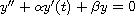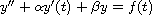Electron. J. Diff. Equ., Vol. 2013 (2013), No. 184, pp. 1-7.

### Hyers-Ulam stability of linear second-order differential equations in complex Banach spaces Yongjin Li, Jinghao Huang

Abstract:
We prove the Hyers-Ulam stability of linear second-order differential equations in complex Banach spaces. That is, if y is an approximate solution of the differential equationor, then there exists an exact solution of the differential equation near to y.

Submitted May 1, 2013. Published August 10, 2013.
Math Subject Classifications: 34K20, 26D10.
Key Words: Hyers-Ulam stability; differential equation.

Show me the PDF file (191 KB), TEX file, and other files for this article.Yongjin Li Department of Mathematics, Sun Yat-Sen University Guangzhou 510275, China email: stslyj@mail.sysu.edu.cn Jinghao Huang Department of Mathematics, Sun Yat-Sen University Guangzhou 510275, China email: hjinghao@mail2.sysu.edu.cn